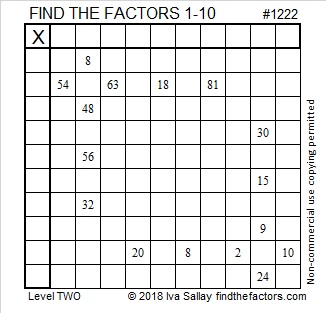# 1222 and Level 2

Can you write the numbers from 1 to 10 in both the first column and the top row of the table below so that the given clues are the multiplication products of the factors you wrote? There is only one solution, but I am sure that you can find it.Print the puzzles or type the solution in this excel file: 10-factors-1221-1231

Now I’ll write a little bit about the number 1222:

• 1222 is a composite number.
• Prime factorization: 1222 = 2 × 13 × 47
• The exponents in the prime factorization are 1, 1, and 1. Adding one to each and multiplying we get (1 + 1)(1 + 1)(1 + 1) = 2 × 2 × 2 = 8. Therefore 1222 has exactly 8 factors.
• Factors of 1222: 1, 2, 13, 26, 47, 94, 611, 1222
• Factor pairs: 1222 = 1 × 1222, 2 × 611, 13 × 94, or 26 × 47
• 1222 has no square factors that allow its square root to be simplified. √1222 ≈ 34.957121222 is the hypotenuse of a Pythagorean triple:
470-1128-1222 which is (5-12-13) times 94

This site uses Akismet to reduce spam. Learn how your comment data is processed.